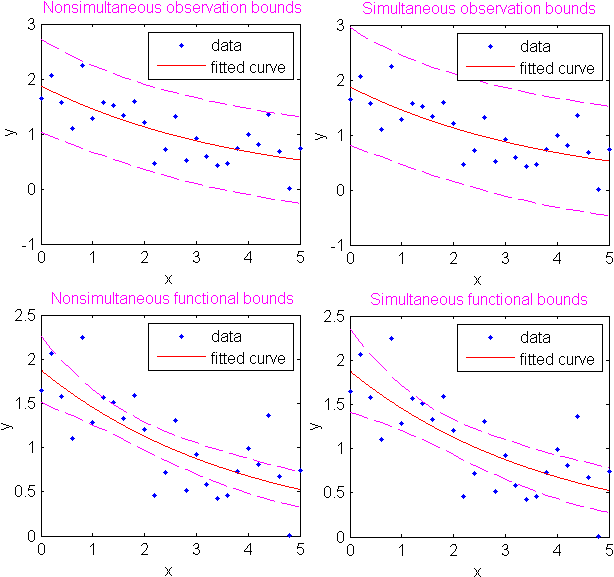Documentation

# polyconf

Polynomial confidence intervals

## Syntax

Y = polyconf(p,X) [Y,DELTA] = polyconf(p,X,S) [Y,DELTA] = polyconf(p,X,S,param1,val1,param2,val2,...) 

## Description

Y = polyconf(p,X) evaluates the polynomial p at the values in X. p is a vector of coefficients in descending powers.

[Y,DELTA] = polyconf(p,X,S) takes outputs p and S from polyfit and generates 95% prediction intervals Y ± DELTA for new observations at the values in X.

[Y,DELTA] = polyconf(p,X,S,param1,val1,param2,val2,...) specifies optional parameter name/value pairs chosen from the following list.

ParameterValue
'alpha'

A value between 0 and 1 specifying a confidence level of 100*(1-alpha)%. The default is 0.05.

'mu'

A two-element vector containing centering and scaling parameters. With this option, polyconf uses (X-mu(1))/mu(2) in place of X.

'predopt'

Either 'observation' (the default) to compute prediction intervals for new observations at the values in X, or 'curve' to compute confidence intervals for the fit evaluated at the values in X. See below.

'simopt'

Either 'off' (the default) for nonsimultaneous bounds, or 'on' for simultaneous bounds. See below.

The 'predopt' and 'simopt' parameters can be understood in terms of the following functions:

• p(x) — the unknown mean function estimated by the fit

• l(x) — the lower confidence bound

• u(x) — the upper confidence bound

Suppose you make a new observation yn+1 at xn+1, so that

yn+1(xn+1) = p(xn+1) + εn+1

By default, the interval [ln+1(xn+1), un+1(xn+1)] is a 95% confidence bound on yn+1(xn+1).

The following combinations of the 'predopt' and 'simopt' parameters allow you to specify other bounds.

'simopt''predopt'Bounded Quantity
'off''observation'

yn+1(xn+1) (default)

'off''curve'

p(xn+1)

'on''observation'

yn+1(x), for all x

'on''curve'

p(x), for all x

In general, 'observation' intervals are wider than 'curve' intervals, because of the additional uncertainty of predicting a new response value (the curve plus random errors). Likewise, simultaneous intervals are wider than nonsimultaneous intervals, because of the additional uncertainty of bounding values for all predictors x.## Examples

This example uses code from the documentation example function polydemo, and calls the documentation example function polystr to convert the coefficient vector p into text for the polynomial expression displayed in the figure title. It combines the functions polyfit, polyval, roots, and polyconf to produce a formatted display of data with a polynomial fit.

### Note

Statistics and Machine Learning Toolbox™ documentation example files are located in the \help\toolbox\stats\examples subdirectory of your MATLAB® root folder (matlabroot). This subdirectory is not on the MATLAB path at installation. To use the files in this subdirectory, either add the subdirectory to the MATLAB path (addpath) or make the subdirectory your current working folder (cd).

Display simulated data with a quadratic trend, a fitted quadratic polynomial, and 95% prediction intervals for new observations:

xdata = -5:5; ydata = xdata.^2 - 5*xdata - 3 + 5*randn(size(xdata)); degree = 2; % Degree of the fit alpha = 0.05; % Significance level % Compute the fit and return the structure used by % POLYCONF. [p,S] = polyfit(xdata,ydata,degree); % Compute the real roots and determine the extent of the % data. r = roots(p)'; % Roots as a row vector. real_r = r(imag(r) == 0); % Real roots. % Assure that the data are row vectors. xdata = reshape(xdata,1,length(xdata)); ydata = reshape(ydata,1,length(ydata)); % Extent of the data. mx = min([real_r,xdata]); Mx = max([real_r,xdata]); my = min([ydata,0]); My = max([ydata,0]); % Scale factors for plotting. sx = 0.05*(Mx-mx); sy = 0.05*(My-my); % Plot the data, the fit, and the roots. hdata = plot(xdata,ydata,'md','MarkerSize',5,... 'LineWidth',2); hold on xfit = mx-sx:0.01:Mx+sx; yfit = polyval(p,xfit); hfit = plot(xfit,yfit,'b-','LineWidth',2); hroots = plot(real_r,zeros(size(real_r)),... 'bo','MarkerSize',5,... 'LineWidth',2,... 'MarkerFaceColor','b'); grid on plot(xfit,zeros(size(xfit)),'k-','LineWidth',2) axis([mx-sx Mx+sx my-sy My+sy]) % Add prediction intervals to the plot. [Y,DELTA] = polyconf(p,xfit,S,'alpha',alpha); hconf = plot(xfit,Y+DELTA,'b--'); plot(xfit,Y-DELTA,'b--') % Display the polynomial fit and the real roots. approx_p = round(100*p)/100; % Round for display. htitle = title(['{\bf Fit: }',... texlabel(polystr(approx_p))]); set(htitle,'Color','b') approx_real_r = round(100*real_r)/100; % Round for display. hxlabel = xlabel(['{\bf Real Roots: }',... num2str(approx_real_r)]); set(hxlabel,'Color','b') % Add a legend. legend([hdata,hfit,hroots,hconf],... 'Data','Fit','Real Roots of Fit',... '95% Prediction Intervals')